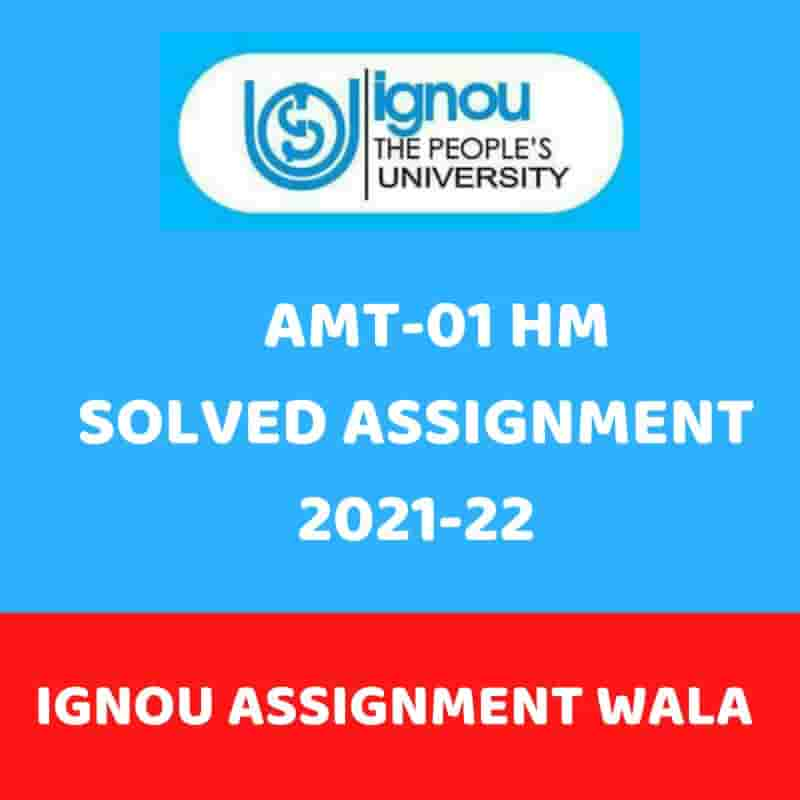## IGNOU AMT-1 HINDI SOLVED ASSIGNMENT 2021-22

Course Code: AMT-01

Assignment Code: AMT-01/TMA/2021-22

Maximum Marks: 100

Note:

• In any question, whenever we ask you to suggest an activity, we expect you to give one other than those covered in the units.
• For any question worth 5 marks, the word limit is about 200 words, for a 10 mark question it is 350 words, and for a 15 mark question it is 500 words.

1. a) Explain how each of the pre-number concepts support the actual process of Your explanation should include an example.                                                                                                              (5)
1. Explain three different ways in which children often regroup the two numbers while undertaking operations on them, using the example of 57 and 38, while solving

57-38.                                                                                                                                     (6)

1. Give an example each of a situation/word problem related to rivers, for the categories below:
1. Cartesian product
2. Augmentation
1. Ratio

Which of these four problems would be the earliest for a child of Class 4 to solve,

and why?                                                                                                                              (9)

1. a) Give two activities, each requiring different ability levels of the learners, to help them understand the concept of ‘negative number’. Justify your choice of activities, also explaining how the second activity requires a higher ability level of the learner

than the first activity.                                                                                                           (7)

1. How would you generalise to any base the concept of place value base 10 and the

process of writing any number using this? How are you using algebra to do this?             (6)

1. After an earthquake, 70 people of the affected community are required to be housed in several Each tent is conical in shape, and must be large enough to allow a family of four to live in it and sleep in it. The radius of the floor space it takes up should be 3 metres, and the tallest human in each family would be around 2 metres. How much material is required to make up the tent?

Solve the problem above, giving the stages involved while doing so.                                   (8)

1. a) Give two distinct word problems (pertaining to the town you stay in) represented by

the expression 2 3 y 1 . Also find the solution to them.                                                       (5)

4     5

1. Is every natural number a fraction? Is every decimal number a fraction? Give

1. Give three errors children commonly make regarding decimal Further, for any one of these errors, give a series of three activities to help a group of children correct the misconception underlying the error. Also explain how each activity

would meet the objective, and how the three activities are connected as a series.                (15)

1. a) Explain the five Van Hiele levels of development of spatial understanding in the

context of measuring shape and size of 2D figures.                                                            (10)

1. Is there a relationship between the perimeter and area of quadrilaterals? Give

1. Give two misconceptions children often have about measurement of (2)
1. a) Give an appropriate example each in support of the statements Also justify

1. Classroom relationships become a resource for developing the mathematical abilities of children.
2. Each child needs time to reflect on the mathematical concept or process being
• Learning experiences should be designed so as to build on existing proficiencies, interests and experiences, for effective mathematics teaching.
1. The ability to make connections between apparently separate mathematical ideas is crucial for conceptual understanding.
 (5)
• Mathematical problems can have diverse
1. b) Through an example pertaining to ‘time’, explain what the diagram below is

(7)

SPECIALISE

CONJECTURE GENERALISE

PROVE/ DISPROOVE